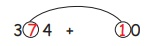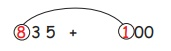Home | | Maths 4th Std | Mental Arithmetic: add and subtract multiples of 10 and 100 mentally

# Mental Arithmetic: add and subtract multiples of 10 and 100 mentally

Mental Arithmetic : Able to add and subtract multiples of 10 and 100 mentally.

Mental Arithmetic

Able to add and subtract multiples of 10 and 100 mentally.

Multiples of 10 and 100 are useful in counting large number of items and objects (including money).

Multiples of 10

10, 20, 30, 40, 50....Here, one’s place didn’t change, when they are added to zero and hundreds Place also didn’t change, only the tens place got changed.

i) 2 + 1 = 3

ii) 4 + 1 = 5

EXAMPLE 14 + 0 = 4

7 + 1 = 8

Therefore 374 + 10 = 384

EXAMPLE 28 + 3 = 11

Here, 11 has two digits, in this keep 1 in the tens place, take the other 1 and add with the hundreds place digit.

Therefore 286 + 30 = 316Here, one's place and ten's place didn't change, when they are added to zero but in the hundreds place it is changed.

EXAMPLE 32 + 0 = 2

8 + 0 = 8

6 + 1 = 7

682 + 100 = 782

EXAMPLE 48 + 1 = 9

835 + 100 = 935

Subtracting by multiples of 10

EXAMPLE 5

Subtract 10 from 6255 – 0 = 5

2 – 1 = 1

625 – 10 = 615

EXAMPLE 6

Subtract 50 from 9818 – 5 = 3

981 – 50 = 931

Subtracting by multiples of 100

EXAMPLE 7

Subtract 400 from 5465 – 4 = 1

546 – 400 = 146

Tags : Numbers | Term 3 Chapter 2 | 4th Maths , 4th Maths : Term 3 Unit 2 : Numbers
Study Material, Lecturing Notes, Assignment, Reference, Wiki description explanation, brief detail
4th Maths : Term 3 Unit 2 : Numbers : Mental Arithmetic: add and subtract multiples of 10 and 100 mentally | Numbers | Term 3 Chapter 2 | 4th Maths